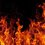# Someone so brave needed.

Hello , everyone , I need help . Does the following have definite value or not , if yes , then what is it ?

$1+\frac{1}{4} +\frac {1}{9} +\frac {1}{16}+\frac{1}{25} . . . \infty$Note by Utkarsh Dwivedi
6 years, 8 months ago

This discussion board is a place to discuss our Daily Challenges and the math and science related to those challenges. Explanations are more than just a solution — they should explain the steps and thinking strategies that you used to obtain the solution. Comments should further the discussion of math and science.

When posting on Brilliant:

• Use the emojis to react to an explanation, whether you're congratulating a job well done , or just really confused .
• Ask specific questions about the challenge or the steps in somebody's explanation. Well-posed questions can add a lot to the discussion, but posting "I don't understand!" doesn't help anyone.
• Try to contribute something new to the discussion, whether it is an extension, generalization or other idea related to the challenge.

MarkdownAppears as
*italics* or _italics_ italics
**bold** or __bold__ bold
- bulleted- list
• bulleted
• list
1. numbered2. list
1. numbered
2. list
Note: you must add a full line of space before and after lists for them to show up correctly
paragraph 1paragraph 2

paragraph 1

paragraph 2

[example link](https://brilliant.org)example link
> This is a quote
This is a quote
    # I indented these lines
# 4 spaces, and now they show
# up as a code block.

print "hello world"
# I indented these lines
# 4 spaces, and now they show
# up as a code block.

print "hello world"
MathAppears as
Remember to wrap math in $$ ... $$ or $ ... $ to ensure proper formatting.
2 \times 3 $2 \times 3$
2^{34} $2^{34}$
a_{i-1} $a_{i-1}$
\frac{2}{3} $\frac{2}{3}$
\sqrt{2} $\sqrt{2}$
\sum_{i=1}^3 $\sum_{i=1}^3$
\sin \theta $\sin \theta$
\boxed{123} $\boxed{123}$

Sort by:

http://en.wikipedia.org/wiki/Basel_problem

- 6 years, 8 months ago

Thanks , Bro. Well, just asking that have you realised it's implications that it has a fixed value ?

- 6 years, 8 months ago

I don't understand what you mean. I don't see any very obvious implication of this fact.

- 6 years, 8 months ago

Not going in too different direction , but , once , when this thing came to my mind then , one of my colleagues got up with an idea.

Here's the idea : Suppose there is a line of massive objects ( perhaps black holes or stars or whatever you may want ) , such that each object touches the adjacent two objects . All objects in the line have a fixed mass, let's say $M$. And they all are arranged in a straight line. They all are spherical with a fixed diameter $d$ and are touching each other. There are infinite objects arranged in a endless line. Remember , they are in absolute space without any influence of any force . What we have to do is with their gravitational force . A simple question : What is the total force acting on each of the objects ( in their center of mass , perhaps ) by the gravity of all the infinite objects on one of its side . My approach :

By Law of Gravitation , total force exerted by all the objects : $\frac {GMM_{1}}{r^{2}} + \frac {GMM_{2}}{(2r)^{2}} + . . . + \frac {GMM_{\infty}}{(nr)^{2}}$ Where mass of our selected object is $M$ . And r is the distance. Now as mass of all object is the same and the distance is or should be a multiple of $d$ , clearly , it is equal to : $\frac {GM^{2}}{d^{2}}(1 +\frac {1}{2^{2}}+\frac {1}{3^{2}} . . .)$ And so as we have both discovered the sum of the infinite sequence is finite or has a fixed value , so we can say the force of gravity would be finite , even though it seems to be infinite . If you haven't understood just try to visualize .

- 6 years, 8 months ago

- 6 years, 8 months ago

@Michael Mendrin , Is my approach correct ?

- 6 years, 8 months ago

Pi^2/6. For a solution you can go to plus.mah.org which is a very good website explaining MATHS and PHYSICS.

- 6 years, 7 months ago

Yeah, I've had that , well, the thing is that , you see, my comment to @Siddhartha Srivastava about the gravity and all , is that true ? , this note was created to understand that concept. Any ideas ?

- 6 years, 7 months ago